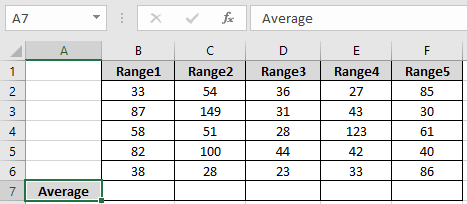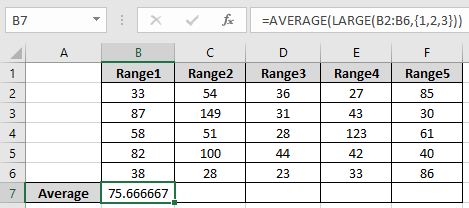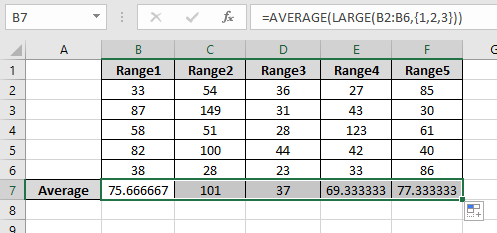# AVERAGE of top 3 scores in a list in excel

In this article, we will learn about how to get the AVERAGE of top 3 scores in a list in excel.

To get the top 3 values in a list we will use the LARGE function.

The LARGE function returns the kth largest value starting from the largest in a range of values.

Syntax:

=LARGE ( array, k )

Note: It will return #NUM! Error if k is greater than the number of values in the range.

AVERAGE function takes the array and returns the average value or mean value for the array.
Syntax:

= AVERAGE ( number1 , number2 ,... )

Let's make a formula using the above functions:
Firstly, we will filter the array using the LARGE function and then the outcome will be fed to the AVERAGE function.
Syntax:

= AVERAGE ( LARGE ( array , { 1 , 2, 3 } ) )

The above formula gets the first largest ,second largest and the third largest value from the array and fed to the AVERAGE function to get the result.

This all might be confusing. So Let’s use the formula in an example to get its more understanding.

Here we have 5 ranges of numbers and We need to find the find the average of the top 3 values for all ranges.We need to find the average using the below formula
Use the formula:

= AVERAGE ( LARGE ( B2:B6 , {1 , 2 , 3 } ) )

Explanation:
The LARGE function gets the top 3 values of the range ( B1:B6 ). The Large function returns the values { 87 , 82 , 58 }. Now AVERAGE of these values is calculated using the AVERAGE functionAs you can see the formula returns the average for first array
Now use the same formula for other ranges using the Ctrl + R or drag right option in excel.Here we have the average for all the ranges.

That's all about, how to get the AVERAGE of top 3 scores in a list in excel. Explore more articles on Excel AVERAGE Formulas here. Please feel free to state your query or feedback for the above article.

Related Articles

Average Difference between lists

Calculate Weighted Average

Ignore zero in the Average of numbers

How to use the AVERAGEIF function in excel

How to use the AVERAGEIFS function in excel

Popular Articles

Edit a dropdown list

If with conditional formatting

If with wildcards

Vlookup by date

Terms and Conditions of use

The applications/code on this site are distributed as is and without warranties or liability. In no event shall the owner of the copyrights, or the authors of the applications/code be liable for any loss of profit, any problems or any damage resulting from the use or evaluation of the applications/code.